# modalfrf

Frequency-response functions for modal analysis

## Syntax

``frf = modalfrf(x,y,fs,window)``
``frf = modalfrf(x,y,fs,window,noverlap)``
``frf = modalfrf(___,Name,Value)``
``````[frf,f,coh] = modalfrf(___)``````
``[frf,f] = modalfrf(sys)``
``frf = modalfrf(sys,f)``
``modalfrf(___)``

## Description

example

````frf = modalfrf(x,y,fs,window)` estimates a matrix of frequency response functions, `frf`, from the excitation signals, `x`, and the response signals, `y`, all sampled at a rate `fs`. The output, `frf`, is an H1 estimate computed using Welch’s method with `window` to window the signals. `x` and `y` must have the same number of rows. If `x` or `y` is a matrix, each column represents a signal.The system response, `y`, is assumed to contain acceleration measurements. To compute a frequency-response function starting from displacement or velocity measurements, use the `'Sensor'` argument. `modalfrf` always outputs the frequency-response function in dynamic flexibility (receptance) format irrespective of the sensor type.```
````frf = modalfrf(x,y,fs,window,noverlap)` specifies `noverlap` samples of overlap between adjoining segments.```

example

````frf = modalfrf(___,Name,Value)` specifies options using name-value arguments, using any combination of inputs from previous syntaxes. Options include the estimator, the measurement configuration, and the type of sensor measuring the system response.```

example

``````[frf,f,coh] = modalfrf(___)``` also returns the frequency vector corresponding to each frequency-response function, as well as the multiple coherence matrix.```
````[frf,f] = modalfrf(sys)` computes the frequency-response function of the identified model `sys`. Use estimation commands like `ssest` (System Identification Toolbox), `n4sid` (System Identification Toolbox), or `tfest` (System Identification Toolbox) to create `sys` from time-domain input and output signals. This syntax allows use only of the `'Sensor'` name-value argument. You must have a System Identification Toolbox™ license to use this syntax.```
````frf = modalfrf(sys,f)` specifies the frequencies at which to compute `frf`. This syntax allows use only of the `'Sensor'` name-value argument. You must have a System Identification Toolbox license to use this syntax.```

example

````modalfrf(___)` with no output arguments plots the frequency response functions in the current figure. The plots are limited to the first four excitations and four responses.```

## Examples

collapse all

Visualize the frequency-response function of a single-input/single-output hammer excitation.

Load a data file that contains:

• `Xhammer` $\text{—}$ An input excitation signal consisting of five hammer blows delivered periodically.

• `Yhammer` $\text{—}$ The response of a system to the input. `Yhammer` is measured as a displacement.

The signals are sampled at 4 kHz. Plot the excitation and output signals.

```load modaldata subplot(2,1,1) plot(thammer,Xhammer(:)) ylabel('Force (N)') subplot(2,1,2) plot(thammer,Yhammer(:)) ylabel('Displacement (m)') xlabel('Time (s)')```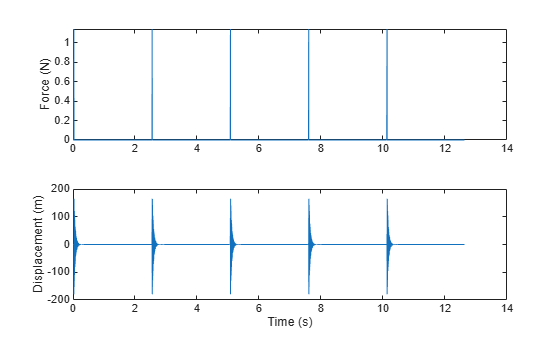Compute and display the frequency-response function. Window the signals using a rectangular window. Specify that the window covers the period between hammer blows.

```clf winlen = size(Xhammer,1); modalfrf(Xhammer(:),Yhammer(:),fs,winlen,'Sensor','dis')```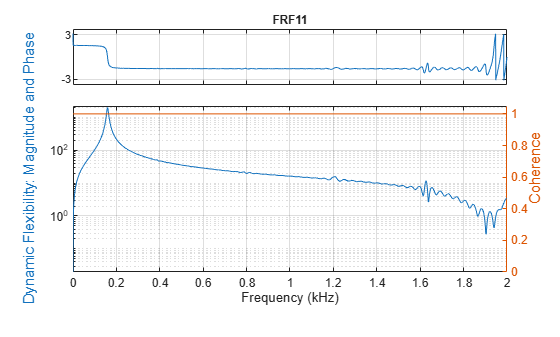Compute the frequency-response functions for a two-input/two-output system excited by random noise.

Load a data file that contains `Xrand`, the input excitation signal, and `Yrand`, the system response. Compute the frequency-response functions using a 5000-sample Hann window and 50% overlap between adjoining data segments. Specify that the output measurements are displacements.

```load modaldata winlen = 5000; frf = modalfrf(Xrand,Yrand,fs,hann(winlen),0.5*winlen,'Sensor','dis');```

Use the plotting functionality of `modalfrf` to visualize the responses.

`modalfrf(Xrand,Yrand,fs,hann(winlen),0.5*winlen,'Sensor','dis')`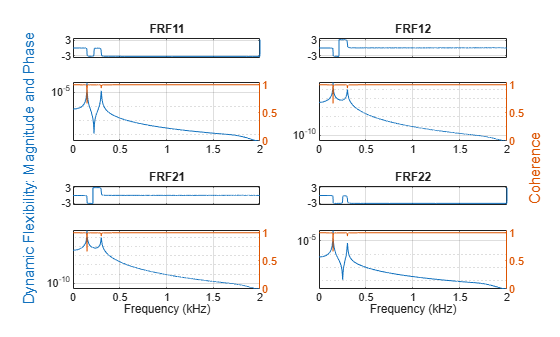Estimate the frequency-response function for a simple single-input/single-output system and compare it to the definition.

A one-dimensional discrete-time oscillating system consists of a unit mass, $\mathit{m}$, attached to a wall by a spring with elastic constant $\mathit{k}=1$. A sensor samples the displacement of the mass at ${\mathit{F}}_{\mathrm{s}}=1$ Hz. A damper impedes the motion of the mass by exerting on it a force proportional to speed, with damping constant $\mathit{b}=0.01$.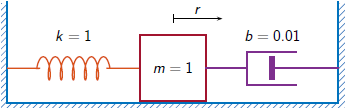Generate 3000 time samples. Define the sampling interval $\Delta \mathit{t}=1/{\mathit{F}}_{\mathit{s}}$.

```Fs = 1; dt = 1/Fs; N = 3000; t = dt*(0:N-1); b = 0.01;```

The system can be described by the state-space model

`$\begin{array}{c}x\left(k+1\right)=Ax\left(k\right)+Bu\left(k\right),\\ y\left(k\right)=Cx\left(k\right)+Du\left(k\right),\end{array}$`

where $\mathit{x}={\left[\begin{array}{cc}\mathit{r}& \mathit{v}\end{array}\right]}^{\mathit{T}}$ is the state vector, $\mathit{r}$ and $\mathit{v}$ are respectively the displacement and velocity of the mass, $\mathit{u}$ is the driving force, and $\mathit{y}=\mathit{r}$ is the measured output. The state-space matrices are

`$A=\mathrm{exp}\left({A}_{c}\Delta t\right),\phantom{\rule{1em}{0ex}}B={A}_{c}^{-1}\left(A-I\right){B}_{c},\phantom{\rule{1em}{0ex}}C=\left[\begin{array}{cc}1& 0\end{array}\right],\phantom{\rule{1em}{0ex}}D=0,$`

$\mathit{I}$ is the $2×2$ identity, and the continuous-time state-space matrices are

`${A}_{c}=\left[\begin{array}{cc}0& 1\\ -1& -b\end{array}\right],\phantom{\rule{1em}{0ex}}{B}_{c}=\left[\begin{array}{c}0\\ 1\end{array}\right].$`

```Ac = [0 1;-1 -b]; A = expm(Ac*dt); Bc = [0;1]; B = Ac\(A-eye(2))*Bc; C = [1 0]; D = 0;```

The mass is driven by random input for the first 2000 seconds and then left to return to rest. Use the state-space model to compute the time evolution of the system starting from an all-zero initial state. Plot the displacement of the mass as a function of time.

```rng default u = randn(1,N)/2; u(2001:end) = 0; y = 0; x = [0;0]; for k = 1:N y(k) = C*x + D*u(k); x = A*x + B*u(k); end plot(t,y)```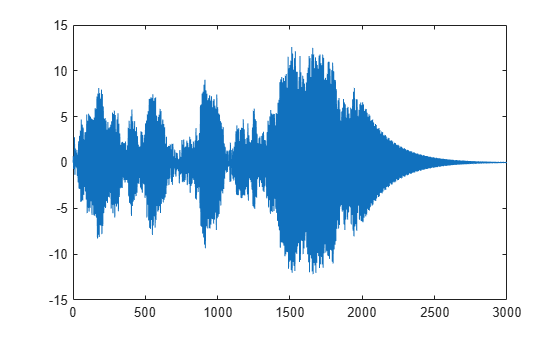Estimate the modal frequency-response function of the system. Use a Hann window half as long as the measured signals. Specify that the output is the displacement of the mass.

```wind = hann(N/2); [frf,f] = modalfrf(u',y',Fs,wind,'Sensor','dis');```

The frequency-response function of a discrete-time system can be expressed as the Z-transform of the time-domain transfer function of the system, evaluated at the unit circle. Compare the `modalfrf` estimate with the definition.

```[b,a] = ss2tf(A,B,C,D); nfs = 2048; fz = 0:1/nfs:1/2-1/nfs; z = exp(2j*pi*fz); ztf = polyval(b,z)./polyval(a,z); plot(f,20*log10(abs(frf))) hold on plot(fz*Fs,20*log10(abs(ztf))) hold off grid ylim([-60 40])```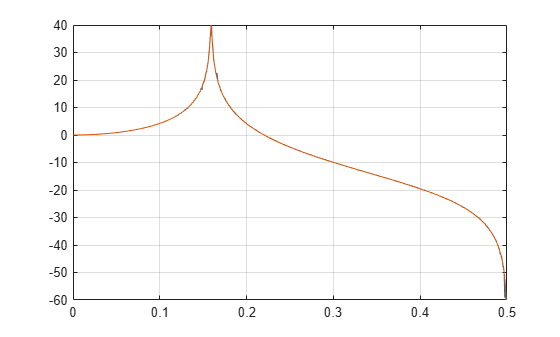Estimate the natural frequency and the damping ratio for the vibration mode.

`[fn,dr] = modalfit(frf,f,Fs,1,'FitMethod','PP')`
```fn = 0.1593 ```
```dr = 0.0043 ```

Compare the natural frequency to $1/2\pi$, which is the theoretical value for the undamped system.

`theo = 1/(2*pi)`
```theo = 0.1592 ```

Estimate the frequency-response function and modal parameters of a simple multi-input/multi-output system.

An ideal one-dimensional oscillating system consists of two masses, ${\mathit{m}}_{1}$ and ${\mathit{m}}_{2}$, confined between two walls. The units are such that ${\mathit{m}}_{1}=1$ and ${\mathit{m}}_{2}=\mu$. Each mass is attached to the nearest wall by a spring with an elastic constant $\mathit{k}$. An identical spring connects the two masses. Three dampers impede the motion of the masses by exerting on them forces proportional to speed, with damping constant $\mathit{b}$. Sensors sample ${\mathit{r}}_{1}$ and ${\mathit{r}}_{2}$, the displacements of the masses, at ${\mathit{F}}_{\mathrm{s}}=50$ Hz.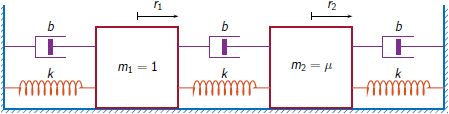Generate 30,000 time samples, equivalent to 600 seconds. Define the sampling interval $\Delta \mathit{t}=1/{\mathit{F}}_{\mathrm{s}}$.

```Fs = 50; dt = 1/Fs; N = 30000; t = dt*(0:N-1);```

The system can be described by the state-space model

`$\begin{array}{c}x\left(k+1\right)=Ax\left(k\right)+Bu\left(k\right),\\ y\left(k\right)=Cx\left(k\right)+Du\left(k\right),\end{array}$`

where $x={\left[\begin{array}{cccc}{r}_{1}& {v}_{1}& {r}_{2}& {v}_{2}\end{array}\right]}^{T}$ is the state vector, ${r}_{i}$ and ${v}_{i}$ are respectively the location and the velocity of the $i$th mass, $u={\left[\begin{array}{cc}{u}_{1}& {u}_{2}\end{array}\right]}^{T}$ is the vector of input driving forces, and $y={\left[\begin{array}{cc}{r}_{1}& {r}_{2}\end{array}\right]}^{T}$ is the output vector. The state-space matrices are

`$A=\mathrm{exp}\left({A}_{c}\Delta t\right),\phantom{\rule{1em}{0ex}}B={A}_{c}^{-1}\left(A-I\right){B}_{c},\phantom{\rule{1em}{0ex}}C=\left[\begin{array}{cccc}1& 0& 0& 0\\ 0& 0& 1& 0\end{array}\right],\phantom{\rule{1em}{0ex}}D=\left[\begin{array}{cc}0& 0\\ 0& 0\end{array}\right],$`

$\mathit{I}$ is the $4×4$ identity, and the continuous-time state-space matrices are

`${A}_{c}=\left[\begin{array}{cccc}0& 1& 0& 0\\ -2k& -2b& k& b\\ 0& 0& 0& 1\\ k/\mu & b/\mu & -2k/\mu & -2b/\mu \end{array}\right],\phantom{\rule{1em}{0ex}}{B}_{c}=\left[\begin{array}{cc}0& 0\\ 1& 0\\ 0& 0\\ 0& 1/\mu \end{array}\right].$`

Set $\mathit{k}=400$, $\mathit{b}=0.1$, and $\mu =1/10$.

```k = 400; b = 0.1; m = 1/10; Ac = [0 1 0 0;-2*k -2*b k b;0 0 0 1;k/m b/m -2*k/m -2*b/m]; A = expm(Ac*dt); Bc = [0 0;1 0;0 0;0 1/m]; B = Ac\(A-eye(4))*Bc; C = [1 0 0 0;0 0 1 0]; D = zeros(2);```

The masses are driven by random input throughout the measurement. Use the state-space model to compute the time evolution of the system starting from an all-zero initial state.

```rng default u = randn(2,N); y = [0;0]; x = [0;0;0;0]; for kk = 1:N y(:,kk) = C*x + D*u(:,kk); x = A*x + B*u(:,kk); end```

Use the input and output data to estimate the transfer function of the system as a function of frequency. Use a 15000-sample Hann window with 9000 samples of overlap between adjoining segments. Specify that the measured outputs are displacements.

```wind = hann(15000); nove = 9000; [FRF,f] = modalfrf(u',y',Fs,wind,nove,'Sensor','dis');```

Compute the theoretical transfer function as the Z-transform of the time-domain transfer function, evaluated at the unit circle.

```nfs = 2048; fz = 0:1/nfs:1/2-1/nfs; z = exp(2j*pi*fz); [b1,a1] = ss2tf(A,B,C,D,1); [b2,a2] = ss2tf(A,B,C,D,2); frf(1,:,1) = polyval(b1(1,:),z)./polyval(a1,z); frf(1,:,2) = polyval(b1(2,:),z)./polyval(a1,z); frf(2,:,1) = polyval(b2(1,:),z)./polyval(a2,z); frf(2,:,2) = polyval(b2(2,:),z)./polyval(a2,z);```

Plot the estimates and overlay the theoretical predictions.

```for jk = 1:2 for kj = 1:2 subplot(2,2,2*(jk-1)+kj) plot(f,20*log10(abs(FRF(:,jk,kj)))) hold on plot(fz*Fs,20*log10(abs(frf(jk,:,kj)))) hold off axis([0 Fs/2 -100 0]) title(sprintf('Input %d, Output %d',jk,kj)) end end```Plot the estimates by using the syntax of `modalfrf` with no output arguments.

```figure modalfrf(u',y',Fs,wind,nove,'Sensor','dis')```Estimate the natural frequencies, damping ratios, and mode shapes of the system. Use the peak-picking method for the calculation.

```[fn,dr,ms] = modalfit(FRF,f,Fs,2,'FitMethod','pp'); fn```
```fn = fn(:,:,1) = 3.8466 3.8466 3.8495 3.8495 fn(:,:,2) = 3.8492 3.8490 3.8552 14.4684 ```

Compare the natural frequencies to the theoretical predictions for the undamped system.

`undamped = sqrt(eig([2*k -k;-k/m 2*k/m]))/2/pi`
```undamped = 2×1 3.8470 14.4259 ```

Compute the frequency-response function of a two-input/six-output data set corresponding to a steel frame.

Load a structure containing the input excitations and the output accelerometer measurements. The system is sampled at 1024 Hz for about 3.9 seconds.

```load modaldata SteelFrame X = SteelFrame.Input; Y = SteelFrame.Output; fs = SteelFrame.Fs;```

Use the subspace method to compute the frequency-response functions. Divide the input and output signals into nonoverlapping, 1000-sample segments. Window each segment using a rectangular window. Specify a model order of 36.

`[frf,f] = modalfrf(X,Y,fs,1000,'Estimator','subspace','Order',36);`

Visualize the stabilization diagram for the system. Identify up to 15 physical modes.

`modalsd(frf,f,fs,'MaxModes',15)`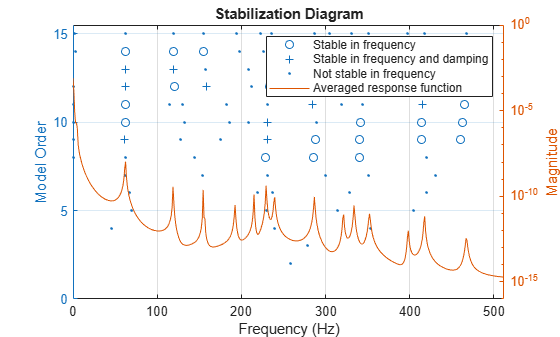## Input Arguments

collapse all

Excitation signals, specified as a vector or matrix.

Data Types: `single` | `double`

Response signals, specified as a vector or matrix.

Data Types: `single` | `double`

Sample rate, specified as a positive scalar expressed in hertz.

Data Types: `single` | `double`

Window, specified as an integer or as a row or column vector. Use `window` to divide the signal into segments:

• If `window` is an integer, then `modalfrf` divides `x` and `y` into segments of length `window` and windows each segment with a rectangular window of that length.

• If `window` is a vector, then `modalfrf` divides `x` and `y` into segments of the same length as the vector and windows each segment using `window`.

• If `'Estimator'` is specified as `'subspace'`, then `modalfrf` ignores the shape of `window` and uses its length to determine the number of frequency points in the returned frequency-response function.

If the length of `x` and `y` cannot be divided exactly into an integer number of segments with `noverlap` overlapping samples, then the signals are truncated accordingly.

For a list of available windows, see Windows.

Example: `hann(N+1)` and `(1-cos(2*pi*(0:N)'/N))/2` both specify a Hann window of length `N` + 1.

Data Types: `single` | `double`

Number of overlapped samples, specified as a positive integer.

• If `window` is a scalar, then `noverlap` must be smaller than `window`.

• If `window` is a vector, then `noverlap` must be smaller than the length of `window`.

Data Types: `double` | `single`

Identified system, specified as a model with identified parameters. Use estimation commands like `ssest` (System Identification Toolbox), `n4sid` (System Identification Toolbox), or `tfest` (System Identification Toolbox) to create `sys` from time-domain input and output signals. See Modal Analysis of Identified Models for an example. Syntaxes that use `sys` typically require less data than syntaxes that use nonparametric methods. You must have a System Identification Toolbox license to use this input argument.

Example: ```idss([0.5418 0.8373;-0.8373 0.5334],[0.4852;0.8373],[1 0],0,[0;0],[0;0],1)``` generates an identified state-space model corresponding to a unit mass attached to a wall by a spring of unit elastic constant and a damper with constant 0.01. The displacement of the mass is sampled at 1 Hz.

Example: `idtf([0 0.4582 0.4566],[1 -1.0752 0.99],1)` generates an identified transfer-function model corresponding to a unit mass attached to a wall by a spring of unit elastic constant and a damper with constant 0.01. The displacement of the mass is sampled at 1 Hz.

Frequencies, specified as a vector expressed in Hz.

Data Types: `single` | `double`

### Name-Value Arguments

Specify optional pairs of arguments as `Name1=Value1,...,NameN=ValueN`, where `Name` is the argument name and `Value` is the corresponding value. Name-value arguments must appear after other arguments, but the order of the pairs does not matter.

Before R2021a, use commas to separate each name and value, and enclose `Name` in quotes.

Example: `'Sensor','vel','Est','H1'` specifies that the input signal consists of velocity measurements and that the estimator of choice is H1.

Estimator, specified as `'H1'`, `'H2'`, `'Hv'`, or `'subspace'`. See Transfer Function for more information about the H1 and H2 estimators.

• Use `'H1'` when the noise is uncorrelated with the excitation signals.

• Use `'H2'` when the noise is uncorrelated with the response signals. In this case, the number of excitation signals must equal the number of response signals.

• Use `'Hv'` to minimize the discrepancy between modeled and estimated response data by minimizing the trace of the error matrix. Hv is the geometric mean of H1 and H2: Hv = (H1H2)1/2

The measurement must be single-input/single-output (SISO).

• Use `'subspace'` to compute the frequency-response functions using a state-space model. In this case, the `noverlap` argument is ignored. This method typically requires less data than nonparametric approaches. See `n4sid` (System Identification Toolbox) for more information.

Presence of feedthrough in state-space model, specified as a logical value. This argument is available only if `'Estimator'` is specified as `'subspace'`.

Data Types: `logical`

Measurement configuration for equal numbers of excitation and response channels, specified as `'fixed'`, `'rovinginput'`, or `'rovingoutput'`.

• Use `'fixed'` when there are excitation sources and sensors at fixed locations of the system. Each excitation contributes to every response.

• Use `'rovinginput'` when the measurements result from a roving excitation (or roving hammer) test. A single sensor is kept at a fixed location of the system. A single excitation source is placed at multiple locations and produces one sensor response per location. The function output ```frf(:,:,i) = modalfrf(x(:,i),y(:,i))```.

• Use `'rovingoutput'` when the measurements result from a roving sensor test. A single excitation source is kept at a fixed location of the system. A single sensor is placed at multiple locations and responds to one excitation per location. The function output ```frf(:,i) = modalfrf(x(:,i),y(:,i))```.

State-space model order, specified as an integer or row vector of integers. If you specify a vector of integers, then the function selects an optimal order value from the specified range. This argument is available only if `'Estimator'` is specified as `'subspace'`.

Data Types: `single` | `double`

Sensor type, specified as `'acc'`, `'vel'`, or `'dis'`.

• `'acc'` — Specifies that the response signal of the system is proportional to acceleration.

• `'vel'` — Specifies that the response signal of the system is proportional to velocity.

• `'dis'` — Specifies that the response signal of the system is proportional to displacement.

`modalfrf` always outputs the frequency-response function in dynamic flexibility (receptance) format irrespective of the sensor type.

#### Example: Undamped Harmonic Oscillator

The motion of a simple undamped harmonic oscillator of unit mass and elastic constant sampled at a rate ${\mathit{f}}_{\mathrm{s}}=1/\Delta \mathit{t}$ is described by the transfer function

$H\left(z\right)=\frac{{N}_{Sensor}\left(z\right)}{1-2{z}^{-1}\mathrm{cos}\Delta t+{z}^{-2}}$,

where the numerator depends on the magnitude being measured:

• Displacement: ${N}_{dis}\left(z\right)=\left({z}^{-1}+{z}^{-2}\right)\left(1-\mathrm{cos}\Delta t\right)$

• Velocity: ${N}_{vel}\left(z\right)=\left({z}^{-1}-{z}^{-2}\right)\mathrm{sin}\Delta t$

• Acceleration: ${N}_{acc}\left(z\right)=\left(1-{z}^{-1}\right)-\left({z}^{-1}-{z}^{-2}\right)\mathrm{cos}\Delta t$

Compute the frequency-response function for the three possible system response sensor types. Use a sample rate of 2 Hz and 30,000 samples of white noise as input.

```fs = 2; dt = 1/fs; N = 30000; u = randn(N,1); ydis = filter((1-cos(dt))*[0 1 1],[1 -2*cos(dt) 1],u); [frfd,fd] = modalfrf(u,ydis,fs,hann(N/2),Sensor="dis"); yvel = filter(sin(dt)*[0 1 -1],[1 -2*cos(dt) 1],u); [frfv,fv] = modalfrf(u,yvel,fs,hann(N/2),Sensor="vel"); yacc = filter([1 -(1+cos(dt)) cos(dt)],[1 -2*cos(dt) 1],u); [frfa,fa] = modalfrf(u,yacc,fs,hann(N/2),Sensor="acc"); loglog(fd,abs(frfd),fv,abs(frfv),fa,abs(frfa)) grid legend(["dis" "vel" "acc"],Location="best")```In all cases, the generated frequency-response function is in a format corresponding to displacement. Velocity and acceleration measurements are first and second time derivatives, respectively, of displacement measurements. The frequency-response functions are equivalent in the range around the natural frequency of the system. Away from the natural frequency, the frequency-response functions differ.

## Output Arguments

collapse all

Frequency-response functions, returned as a vector, matrix, or 3-D array. `frf` has size p-by-m-by-n, where p is the number of frequency bins, m is the number of responses, and n is the number of excitation signals.

`modalfrf` always outputs the frequency-response function in dynamic flexibility (receptance) format irrespective of the sensor type.

Frequencies, returned as a vector.

Multiple coherence matrix, returned as a matrix. `coh` has one column for each response signal.

 "Dynamic Stiffness, Compliance, Mobility, and more..." Siemens, last modified 2019, https://community.sw.siemens.com/s/article/dynamic-stiffness-compliance-mobility-and-more.

 Brandt, Anders. Noise and Vibration Analysis: Signal Analysis and Experimental Procedures. Chichester, UK: John Wiley & Sons, 2011.

 Irvine, Tom. "An Introduction to Frequency Response Functions," Vibrationdata, 2000, https://vibrationdata.com/tutorials2/frf.pdf.

 Vold, Håvard, John Crowley, and G. Thomas Rocklin. "New Ways of Estimating Frequency Response Functions." Sound and Vibration. Vol. 18, November 1984, pp. 34–38.